# MECHANISM OF EXPLOSION-INDUCED DISTURBANCE IN NATURAL MAGNETIC FIELD1)

Li Jianqiao, Ma Tianbao, Ning Jianguo2)State Key Laboratory of Explosion Science and Technology,Beijing Institute of Technology, Beijing 100081, China

Abstract

Electromagnetic effect generated by explosions has a significant influence on the explosion test. Some metallized explosives even disable the electronic equipment. The mechanism and numerical simulations are investigated to the magnetic disturbance of natural magnetic field observed during the conventional explosion for getting the theoretical model and the properties of the explosion-induced electromagnetic effect. The mechanism of the generated magnetic disturbance is proposed based on a thermodynamic equilibrium ionization model and the magnetic hydrodynamic (MHD) model considering magnetic diffusion. The magnetic disturbance is considered to be induced by the motion of the conductive detonation products in the geomagnetic field. The conductivity, which makes direct influence on the disturbance duration, is described by the ionization model. Combined with the MHD governing equations and the method utilized in explosion issues, two kinds of numerical simulations are performed to investigate the magnetic disturbance during explosions of rectangular explosive in different initial magnetic fields. It is found that the magnetic disturbances are extremely different in value while almost the same in distribution. The distributions of fluid and magnetic parameters are compared at the same time and a conclusion can be obtained that the same distribution of conductivity leads to a similar distribution of magnetic field. The conductivity of detonation products has a huge difference in space distribution and this makes the magnetic field diffused except in the region with high conductivity. Therefore, obvious magnetic disturbance can be only observed in the region of high conductivity. The difference of magnetic field in value is related to both the initial magnetic field and velocity of fluid and can be only extremely observed in the early stage of explosion. A magnetic disturbance induced by the electromagnetic wave generated in the explosion is simulated and compared with the result in reference. The comparison shows a good agreement in the time duration scale of the magnetic disturbance, which proves the reliability of the numerical method used in this paper. The influence of the configuration of explosives in the geomagnetic field on the magnetic disturbance is first considered and the difference in the magnetic disturbance is predicted. The above achievement has not been reported in the previous published investigations. The prediction proposed in this paper needs a further experimental verification and this will be the next stage of our investigation.

Keywords： plasmas ; non-ideal MHD ; explosion ; magnetic disturbance ; numerical simulation

0

Li Jianqiao, Ma Tianbao, Ning Jianguo. MECHANISM OF EXPLOSION-INDUCED DISTURBANCE IN NATURAL MAGNETIC FIELD1)[J]. Acta Mechanica Sinica, 2018, 50(5): 1206-1218 https://doi.org/10.6052/0459-1879-18-081

## 1 理论模型

### 1.1 爆炸过程热电离模型

\begin{equation*}\text A\rightleftharpoons \text A^++\text e^-\tag*{(1)}\end{equation*}

\begin{equation*}\delta F=\dfrac{\partial F \partial N_0}\delta N_0+\dfrac{\partial F}{\partial N_1}\delta N_1+\dfrac{\partial F}{\partial N_{\text e}}\delta N_{\text e}=0\tag*{(2)}\end{equation*}

\begin{equation*}\delta N_0=-\delta N_1=-\delta N_{\text e}\tag*{(3)}\end{equation*}

\begin{equation*}\dfrac{\partial F}{\partial N_0}-\dfrac{\partial F}{\partial N_1}-\dfrac{\partial F}{\partial N_{\text e}}=0\tag*{(4)}\end{equation*}

\begin{equation*}F=-kT\text{ln}Z\tag*{(5)}\end{equation*}

\begin{equation*}\left.\begin{split}&U_{\text p0}\left(r_1,r_2,\cdots,r_{N_0}\right)=N_0E_{\text p}\\&U_{\text p1}\left(r_1,r_2,\cdots,r_{N_1}\right)=N_1\left(E_{\text p}+I_1\right)\end{split}\right\}\tag*{(7)}\end{equation*}

$E_\text p$表示一个原子或离子所具有的势能.

\begin{equation*}\left.\begin{split}&F=-kT\text{ln}\dfrac{Q_{N_0}Q_{N_1}Q_{N_\text e}}{\lambda^{3(N_1+N_0)}\lambda^{3N_\text e}_\text e N_0!N_1!N_\text e!}\\&\lambda^3=\left\lgroup \dfrac{2\pi \hbar^2}{m_\text akT}\right\rgroup^{\tfrac{3}{2}}\\&\lambda^3_\text e=\left\lgroup \dfrac{2\pi \hbar^2}{m_\text ekT}\right\rgroup^{\tfrac{3}{2}}\end{split}\right\}\tag*{(9)}\end{equation*}

\begin{equation*}\begin{split}&F=kTN\text{ln}\dfrac{\lambda^3_T}{V}+kTN_0\text{ln}N_0-kTN+\\&kTN_1\text{ln}N_1+E_\text pN+I_1N_1+\\&kTN_\text e\text{ln}\dfrac{\lambda^3_{T_e}}{V}+kTN_\text e\text{ln}N_\text e-kTN_{\text e}\end{split}\tag*{(10)}\end{equation*}

\begin{equation*}\left\lgroup\dfrac{m_{\text e}kT}{2\pi h^2} \right\rgroup^{\tfrac{3}{2}}\text{exp}\left\lgroup -\dfrac{I_1}{kT}\right\rgroup=\dfrac{n_{\text e}n_1}{n_0}\tag*{(11)}\end{equation*}

\begin{equation*}\dfrac{\alpha^2}{1-\alpha}=\dfrac{1}{n}\left\lgroup\dfrac{m_\text ekT}{2\pi h^2}\right\rgroup^{\tfrac{3}{2}}\text{exp}\left\lgroup-\dfrac{I_1}{kT}\right\rgroup\tag*{(12)}\end{equation*}

\begin{equation*}\sigma=\dfrac{e^2n_\text e}{\bar{Z}m_\text e},~\bar{Z}=\sqrt{2}\pi d^2n\bar{v},~\bar{v}=\sqrt{\dfrac{3kT}{\pi m_{\text e}}}\tag*{(13)}\end{equation*}

### 1.2 MHD模型

\begin{equation*}\dfrac{\partial}{\partial t}\begin{bmatrix}\rho\\\rho U\\ B\\\rho e_{\text{MHD}}\end{bmatrix}+\nabla\cdot\begin{bmatrix}\rho U\\\rho{UU}+p_{\text{MHD}} I-\dfrac{{BB}}{\mu_\text e}\\{UB}-{BU}\\(\rho e_{\text{MHD}}+p_{\text{MHD}}) U-\dfrac{ B}{\mu_{\text e}}( U\cdot B)-{\tau}\cdot U\end{bmatrix}+\nabla\cdot\begin{bmatrix}0\\-{\tau}\\-v_\text e\nabla B\\0\end{bmatrix}=-(\nabla\cdot B)\begin{bmatrix}0\\\dfrac{ B}{\mu_\text e}\\ U\\ U\cdot\dfrac{ B}{\mu_\text e}\end{bmatrix}\tag*{(15)}\end{equation*}

(1)在不考虑磁扩散的情况下进行对流问题的求解, 这时方程化为

\begin{equation*}\dfrac{\partial}{\partial t}\begin{bmatrix}\rho\\\rho U\\ B\\\rho e_{\text{MHD}}\end{bmatrix}+\nabla\cdot\begin{bmatrix}\rho U\\\rho{UU}+p_{\text{MHD}} I-\dfrac{{BB}}{\mu_\text e}\\{UB}-{BU}\\(\rho e_{\text{MHD}}+p_{\text{MHD}}) U-\dfrac{ B}{\mu_{\text e}}( U\cdot B)\end{bmatrix}+\nabla\cdot\begin{bmatrix}0\\-{\tau}\\\text{\textbf{0}}\\-{\tau}\cdot U\end{bmatrix}=-(\nabla\cdot B)\begin{bmatrix}0\\\dfrac{ B}{\mu_\text e}\\ U\\ U\cdot\dfrac{ B}{\mu_\text e}\end{bmatrix}\tag*{(16)}\end{equation*}

(2)仅考虑磁场的扩散过程, 磁扩散是由于电流密度表达式中电场项引起的, 磁扩散方程原始形式为

\begin{equation*}\dfrac{\partial B}{\partial t}=-\nabla\times(v_\text e\nabla\times B)\tag*{(17)}\end{equation*}

\begin{equation*}\nabla\times(\nabla\times B)=\nabla(\nabla\cdot B)-\nabla\cdot(\nabla B)\tag*{(18)}\end{equation*}

\begin{equation*}\dfrac{\partial B}{\partial t}=-v_\text e(\nabla(\nabla\cdot B)-\nabla\cdot(\nabla B))\tag*{(19)}\end{equation*}

\begin{equation*}\dfrac{\partial B}{\partial t}-\nabla\cdot(v_{\text e}\nabla B)=\text{\textbf{0}}\tag*{(20)}\end{equation*}

## 2 数值模拟

\begin{equation*}p=(\gamma-1)\rho e\tag*{(21)}\end{equation*}

Table 1   Material properties in numerical simulation

Materialρ0/(kg • m-3)E0/(J • kg-1)γT0/Kμe/(T • m • A-1)
explosive16407.0 x 1061.4300.01.257 x 10-6
air1.29196 3661.4300.01.257 x 0-6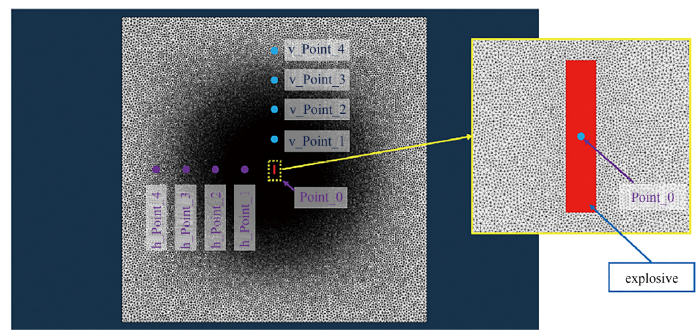Fig.1   The model and mesh used in numerical simulation

Table 2   Initial condition of magnetic field

Simulation caseBx0/GsBy0 /Gs
10.50
200.5

### 2.1 数值计算格式

\begin{equation*}\dfrac{\partial X \partial t}+\nabla\cdot F+\nabla\cdot G= 0\tag*{(22)} \end{equation*}

\begin{equation*}\begin{split}&F=\Bigg\lbrack \rho U,\rho {UU}+p_{\text{MHD}}I-\dfrac{ {BB}}{\mu_\text e}, {UB}- {BU},\\&\quad\quad(\rho e_{\text{MHD}}+p_{\text{MHD}}) U-\dfrac{ B}{\mu_\text e}( U\cdot B)\Bigg\rbrack\end{split}\tag*{(23)}\end{equation*}

$G=[ 0,0\cdot I,-v_\text e\nabla B, 0]$与$F$同阶, 表示黏性通量, 梯度算符$\nabla$ 为行向量, 这样控制方程由式(22) 表示, 将散度进行空间降维, 式(22) 表示为式(24)

\begin{equation*}\iiint\limits_{\varOmega}\dfrac{\partial X}{\partial t}\text d\varOmega+\iint\limits_S n^\text T\cdot F\text dS+\iint\limits_S n^\text T\cdot G\text d S= 0\tag*{(24)}\end{equation*}

$n^\text T$表示微元面的外法向量转置, 有限体积法对于具有$m$个面的单元$i$来说, 其离散的格心格式为

\begin{equation*}\dfrac{\partial X_i}{\partial t}=-\dfrac{1}{\varOmega}\sum\limits^m_{o=1} n^\text T_o\cdot( F_o+ G_o)\cdot S_o\tag*{(25)}\end{equation*}

\begin{align}&\dfrac{\partial X_{i1}}{\partial t}=-\dfrac{1}{\varOmega_i}\sum\limits^{m}_{o=1} n^\text T_o\cdot F_o\cdot S_o\tag*{(26)}\\&\dfrac{\partial X_i}{\partial t}=-\dfrac{1}{\varOmega_i}\sum\limits^m_{o=1} n^\text T_o\cdot G_o\left( X_{i1}\right)\cdot S_o\tag*{(27)}\end{align}

\begin{align*}& F= F^\text c+ F^\text p+ F_\text{MHD}\tag*{(28)}\\& F^\text c=[\rho U,\rho {UU}, {UB},\rho e_\text{MHD} U]\tag*{(29)}\\& F^\text p=[ 0,p_\text{MHD} I, 0,p_\text{MHD} U]\tag*{(30)}\\& F^\text {MHD}=\Bigg[ 0,-\dfrac{ {BB}}{\mu_\text e},- {BU},-\dfrac{ B}{\mu_\text e}( U\cdot B)\Bigg]\tag*{(31)}\\\end{align*}

\begin{equation*}\left.\begin{split}& F_o= F^\text c_o+ F^\text p_o+ F^\text {MHD}_o\\& F^\text p_o=[ 0,p_o I,p_o U_o],~ U_o=\dfrac{1}{2}( U_\text R+ U_\text L) & F^\text c_o= n\cdot F_\text c\\& F_\text c=\begin{cases}a_oM_o X_\text L,M_o>0\\a_oM_o X_\text R,M_o\leqslant0\end{cases}\end{split}\right\}\tag*{(32)}\end{equation*}

### 2.2 背景磁场沿$x$方向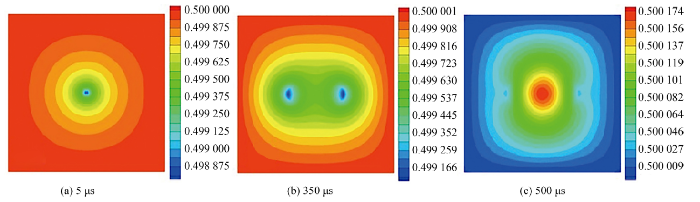Fig.2   Numerical simulation of Bx at different time

### 2.3 背景磁场沿$y$方向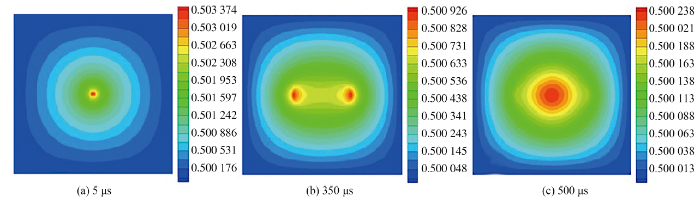Fig.3   Numerical simulation of $B_y$ at different time

## 3 讨论分析Fig.4   Comparison between numerical simulations with different magnetic directions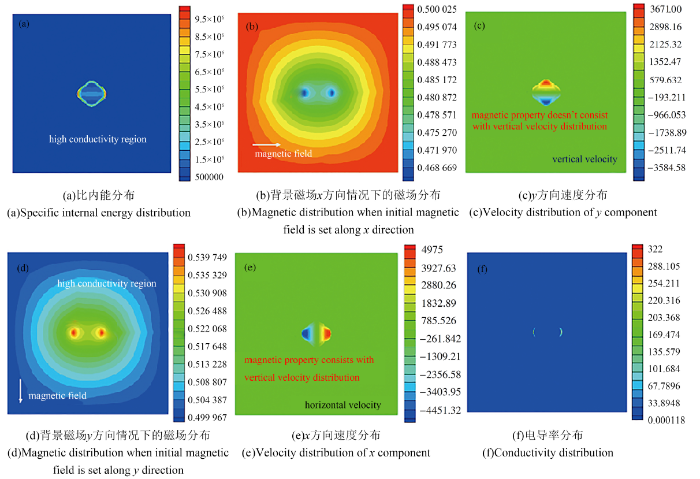Fig.5   Numerical results at 150 μs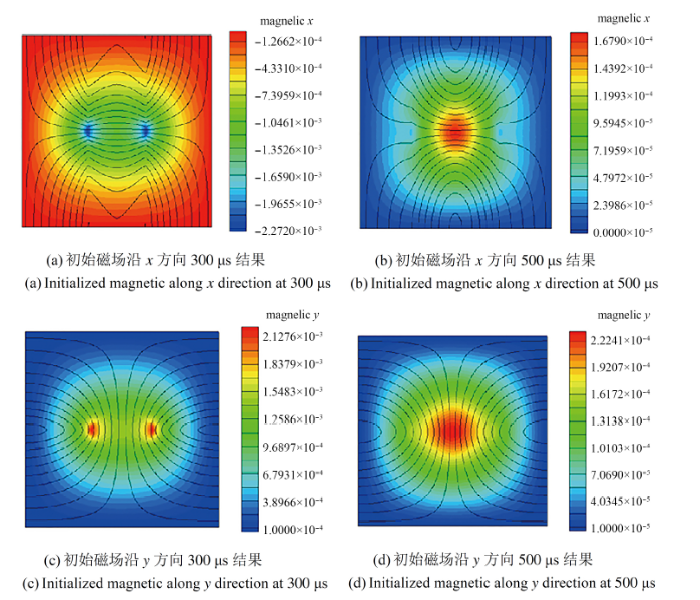Fig.6   Comparison between numerical simulations with different magnetic directions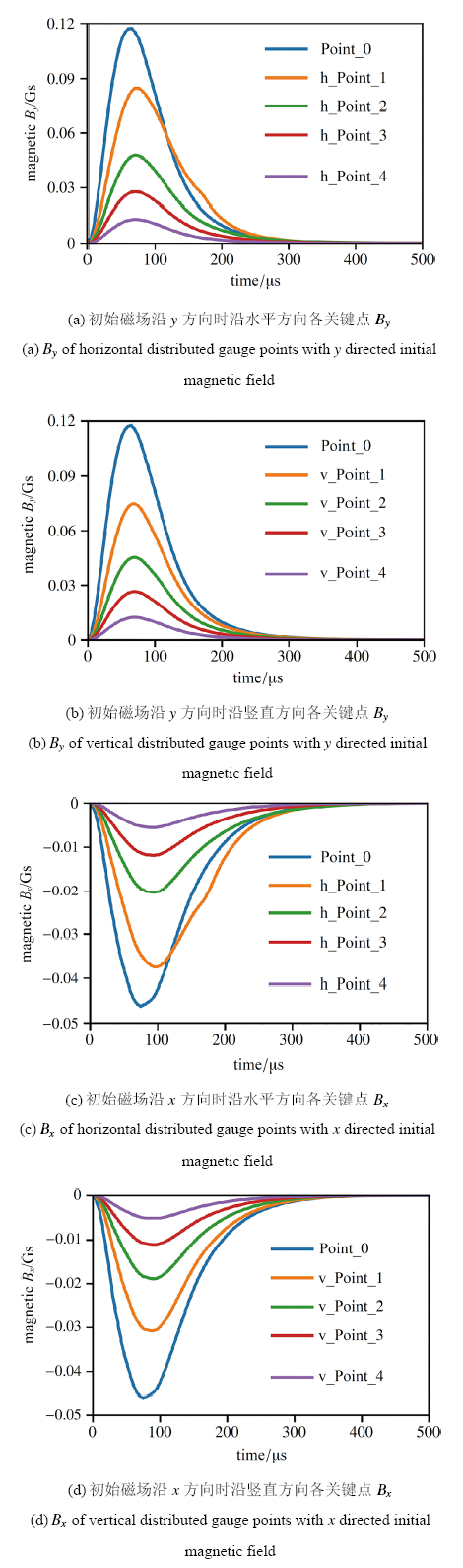Fig.7   Magnetic field history curves on gauge points marked in Fig.1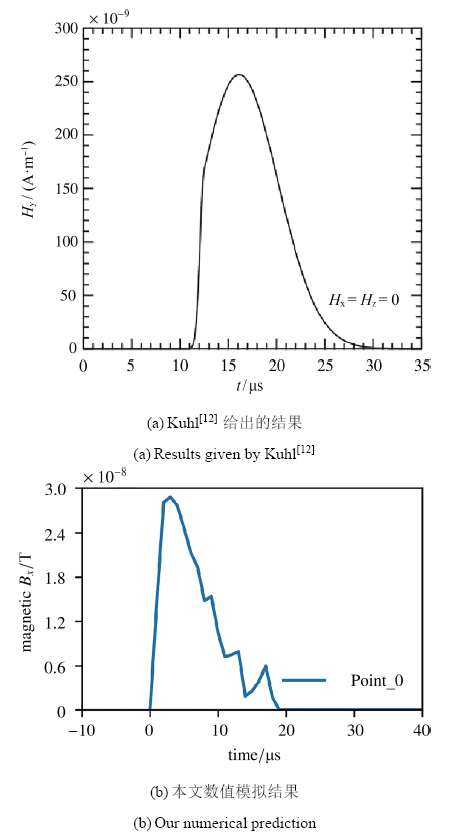Fig.8   Comparison with citation

## 4 结 论

(1) 基于本文提出的机理, 可以获得爆炸过程中自然磁场扰动的结果;

(2) 几何不对称的炸药在摆放方向不同的时候, 爆炸产生的磁场扰动幅值将会有很大的不同, 这一点在目前已有的研究中完全被忽略了, 甚至没有人提出过这种可能性;

(3) 虽然两种工况模拟得到的磁场扰动幅值不同, 但整体的扰动模式是相同的, 主要表现在磁场扰动的空间分布上;

(4) 相同的空间电导率分布导致了不同工况下得到的磁场扰动模式相同.

The authors have declared that no competing interests exist.

## 参考文献 原文顺序 文献年度倒序 文中引用次数倒序 被引期刊影响因子

  戴晴, 李传胪, 陈国强等. 低温等离子体激励宽带电磁波信号的实验研究. 电子信息对抗技术, 2009, 24(5): 72-74 (Dai Qing, Li Chuanlu, Chen Guoqiang, et al.Experimental study of wideband electromagnetic radiation from plasma cloud. Electronic Information Warfare Technology, 2009, 24(5): 72-74 (in Chinese))  Kolsky H.Electromagnetic waves emitted on detonation of explosives. Nature, 1954, 173: 77  Cook MA.The Science of High Explosives. New York: Reinhold Publishing, 1958: 440  Boronin AP, Velmin VA, Medvedev YA, et al.Experimental study of the electromagnetic field in the near zone of explosions produced by solid explosives. Zhurnal Prikladnoi Mekhaniki I Tekhnicheskoi Fiziki, 1968, 9(6): 99-103  Boronin AP, Kapinos VN, Krenev SA, et al.Physical mechanism of electromagnetic field generation during the explosion of condensed explosive charges. Combustion Explosion and Shock Waves, 1990, 26: 597-602  Walker CW. Observations of the electromagnetic signals from high explosive detonation. Lawrence Radiation Laboratory Report, 1970, UCRL-72150  van Lint VAJ. Electromagnetic emission from chemical explosions. IEEE Transactions on Nuclear Science, 1982, NS-29(6): 1844-1849  Soloviev SP, Surkov VV, Sweeney JJ.Quadrupolar electromagnetic field from detonation of high explosive charges on the ground surface. Journal of Geophysical Research, 2002, 107: B62119  Adushkin VV, Soloviev SP.Generation of electric and magnetic fields by area, surface, and underground explosions. Combustion Explosion and Shock Waves, 2004, 40(6): 649-657  Soloviev SP, Sweeney JJ.Generation of electric and magnetic field during detonation of high explosive charges in boreholes. Journal of Geophysical Research, 2005, 110: B01312  Fine JE. Estimates of the electromagnetic radiation from detonation of conventional explosives. Army Research Laboratory Report, 2001, ARL-TR-2447  Kuhl AL, White DA, Kirkendall BA.Kirkendall. Electromagnetic waves from TNT explosions. Journal of Electromagnetic Analysis and Applications, 2014, 6: 280-295  Srnka LJ.Spontaneous magnetic field generation in hypervelocity impacts//Lunar & Planetary Science Conference, 1977, 8: 785-792  陈生玉, 孙新利, 钱世平等. 化爆引起的电磁辐射. 爆炸与冲击, 1997, 17(4): 363-368 (Chen Shengyu, Sun Xinli, Qian Shiping, et al.Electromagnetic radiation caused by chemical explosion. Explosion and Shock Waves, 1997, 17(4): 363-368 (in Chinese))  曹景阳, 谢树果, 苏东林等. 航天火工品爆炸引起的电磁干扰测量. 北京航空航天大学学报, 2011, 37(11): 1384-1394 (Cao Jingyang, Xie Shuguo, Su Donglin, et al.Electromagnetic interference caused by aerospace explosives. Journal of Beijing University of Aeronautics and Astronautics, 2011, 37(11): 1384-1394 (in Chinese))  王长利, 李迅, 刘晓新等. 典型炸药爆炸过程的电磁辐射实验研究. 兵工学报, 2014, 35(S2): 188-192 (Wang Changli, Li Xun, Liu Xiaoxin, et al.The experimental research on the electromagnetic radiation aroused by detonation of explosive. Acta Armamentarii, 2014, 35(S2): 188-192 (in Chinese))  陈鸿, 何勇, 潘绪超等. 铝添加物对炸药爆轰过程中的电磁辐射影响实验研究. 科学发现, 2016, 4(6): 398-404 (Chen Hong, He Yong, Pan Xuchao, et al.Experimental research on the effect of aluminum additive on the electromagnetic radiation in detonation process. Science Discovery, 2016, 4(6): 398-404 (in Chinese))  Eichhorn G.Measurements of the light flash produced by high velocity particle impact. Planetary and Space Science, 1975, 23(11): 1519-1525  Lee N, Close S, Goel A, et al. Theory and experiments characterizing hypervelocity impact plasmas on biased spacecraft materials. Physics of Plasmas, 2013, 20(3): 032901  唐恩凌, 张庆明, 张健. 超高速碰撞LY12铝靶产生膨胀等离子体云的电导率测量. 强激光与粒子束, 2009, 21(2): 297-300 (Tang Enling, Zhang Qingming, Zhang Jian.Conductivity measurement of an expanding plasma cloud generated by hypervelocity impact LY12 aluminum target. High Power Laser and Particle Beams, 2009 21(2): 297-300 (in Chinese))  Lin L, Weng CS, Chen QZ, et al.Study on the effects of ionization seeds on pulse detonation characteristics. Aerospace Science and Technology, 2017, 71: 128-135  王年华, 张来平, 赵钟等. 基于制造解的非结构二阶有限体积离散格式的精度测试与验证. 力学学报, 2017, 49(3): 627-637 (Wang Nianhua, Zhang Laiping, Zhao Zhong, et al.Accuracy verification of unstructured second-order finite volume discretization schemes based on the method of manufactured solutions. Chinese Journal of Theoretical and Applied Mechanics, 2017, 49(3): 627-637 (in Chinese))  马天宝, 任会兰, 李健等. 爆炸与冲击问题的大规模高精度计算. 力学学报, 2016, 48(3): 599-608 (Ma Tianbao, Ren Huilan, Li Jian, et al.Large scale high precision computation for explosion and impact problems. Chinese Journal of Theoretical and Applied Mechanics, 2016, 48(3): 599-608 (in Chinese))  宁建国, 原新鹏, 马天宝等. 计算动力学中的伪弧长方法研究. 力学学报, 2017, 49(3): 703-715 (Ning Jianguo, Yuan Xinpeng, Ma Tianbao, et al.Pseudo arc-length numerical algorithm for computational dynamics. Chinese Journal of Theoretical and Applied Mechanics, 2017, 49(3): 703-715 (in Chinese))  张树海. 加权紧致格式与WENO格式的比较研究. 力学学报, 2016, 48(2): 336-347 (Zhang Shuhai.The comparison of weighted compact schemes and WENO scheme. Chinese Journal of Theoretical and Applied Mechanics, 2016, 48(2): 336-347 (in Chinese))  李海燕, 李志辉, 吕治国等. 自由活塞压缩管ALE方法数值模拟. 力学学报, 2016, 48(2): 348-352 (Li Haiyan, Li Zhihui, Lü Zhiguo, et al.Arbitrary Lagrangian Eulerian simulation of free piston compression tube. Chinese Journal of Theoretical and Applied Mechanics, 2016, 48(2): 348-352 (in Chinese))  Kitamura K, Shima E.Pressure-equation-based SLAU2 for oscilla-tion-free, supercritical flow simulations. Computers and Fluids, 2018, 163: 86-96  Zeng QL, Aydemir NU, Lien FS, et al.Extension of staggered-grid-based AUSM-family schemes for use in nuclear safety analysis codes. International Journal of Multiphase Flow, 2017, 93: 17-32  Qu F, Sun D, Zou G, et al.An improvement on the AUSMPWM scheme for hypersonic heating predictions. International Journal of Heat and Mass Transfer, 2017, 108: 2492-2501  Min Daeho KH, Lee H, Lee CG, et al.Accurate and efficient computations of phase-changing flows in thermal vapor compressors. Applied Thermal Engineering, 2018, 128: 320-334  Gao ZX, Xue HC, Zhang ZC, et al.Ahybrid numerical scheme for aeroheating computation of hypersonic reentry vehicles. International Journal of Heat and Mass Transfer, 2018, 116: 432-444  Qu F, Sun D, Yan C.A new flux splitting scheme for the Euler equations II: E-AUSMPWAS for all speeds. Commun Nonlinear Sci Numer Simulat, 2018, 57: 58-79  Paillere H, Corre C, Cascalse JRG.On the extension of the AUSM+ scheme to compressible two-fluid models. Computers and Fluids, 2003, 32: 891-916  Phongthanapanich S.A parameter-free AUSM-based scheme for healing carbuncle phenomenon. Journal of the Brazilian Society of Mechanical Sciences and Engineering, 2016, 38(3): 691-701  Zeng QL, Aydemir NU, Lien FS, et al.Comparison of implicit and explicit AUSM-family schemes for compressible multiphase flows. International Journal for Numerical Methods in Fluids, 2015, 77: 43-61  Liou MS.A sequel to AUSM, Part II: AUSM+-up for all speeds. Journal of Computational Physics, 2006, 214: 137-170  Zha GC, Bilgen E.Numerical simulations of Euler equations by using a new flux splitting scheme. International Journal for Numerical Method in Fluids, 1993, 17: 115-144  刘成, 姜秀杰, 刘波等. 大气电导率测量技术的发展. 科技导报, 2010, 28(17): 95-99 (Liu Cheng, Jiang Xiujie, Liu Bo, et al.Progress of atmospheric electrical conductivity measurement technology. Science & Technology Review, 2010, 28(17): 95-99 (in Chinese))/

 〈〉